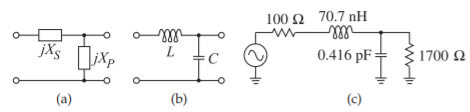# 6.4: The L Matching Network

$$\newcommand{\vecs}{\overset { \rightharpoonup} {\mathbf{#1}} }$$ $$\newcommand{\vecd}{\overset{-\!-\!\rightharpoonup}{\vphantom{a}\smash {#1}}}$$$$\newcommand{\id}{\mathrm{id}}$$ $$\newcommand{\Span}{\mathrm{span}}$$ $$\newcommand{\kernel}{\mathrm{null}\,}$$ $$\newcommand{\range}{\mathrm{range}\,}$$ $$\newcommand{\RealPart}{\mathrm{Re}}$$ $$\newcommand{\ImaginaryPart}{\mathrm{Im}}$$ $$\newcommand{\Argument}{\mathrm{Arg}}$$ $$\newcommand{\norm}{\| #1 \|}$$ $$\newcommand{\inner}{\langle #1, #2 \rangle}$$ $$\newcommand{\Span}{\mathrm{span}}$$ $$\newcommand{\id}{\mathrm{id}}$$ $$\newcommand{\Span}{\mathrm{span}}$$ $$\newcommand{\kernel}{\mathrm{null}\,}$$ $$\newcommand{\range}{\mathrm{range}\,}$$ $$\newcommand{\RealPart}{\mathrm{Re}}$$ $$\newcommand{\ImaginaryPart}{\mathrm{Im}}$$ $$\newcommand{\Argument}{\mathrm{Arg}}$$ $$\newcommand{\norm}{\| #1 \|}$$ $$\newcommand{\inner}{\langle #1, #2 \rangle}$$ $$\newcommand{\Span}{\mathrm{span}}$$

The examples in the previous two sections suggest the basic concept behind lossless matching of two different resistance levels using an $$\text{L}$$ network:Figure $$\PageIndex{1}$$: Parallel-to-series transformation: (a) resistor with shunt capacitor; (b) its equivalent series circuit; and (c) the transforming circuit with added series inductor.Figure $$\PageIndex{2}$$: $$\text{L}$$ matching networks consisting of one shunt reactive element and one series reactive element. ($$R_{S}$$ is matched to $$R_{L}$$.) $$X_{C}$$ is the reactance of the capacitor $$C$$, and $$X_{L}$$ is the reactance of the inductor $$L$$. Note that with a two-element matching network the $$Q$$ and thus bandwidth of the match is fixed.

Step 1: Use a series (shunt) reactive element to transform a smaller (larger) resistance up (down) to a larger (smaller) value with a real part equal to the desired resistance value.

Step 2: Use a shunt (series) reactive element to resonate with (or cancel) the imaginary part of the impedance that results from Step 1.

So a resistance can be transformed to any resistive value by using an $$LC$$ transforming circuit.

Formalizing the matching approach described above, note that there are four possible two-element $$\text{L}$$ matching networks (see Figure $$\PageIndex{2}$$). The two possible cases, $$R_{S} < R_{L}$$ and $$R_{L} < R_{S}$$, will be considered in the following subsections.Figure $$\PageIndex{3}$$: Two-element matching network topology for $$R_{S} < R_{L}$$. $$X_{S}$$ is the series reactance and $$X_{P}$$ is the parallel reactance.

## 6.4.1 Design Equations for $$R_{S}<R_{L}$$

Consider the matching network topology of Figure $$\PageIndex{3}$$. Here

$\label{eq:1}Z_{\text{in}}=\frac{R_{L}(\jmath X_{P})}{R_{L}+\jmath X_{P}}=\frac{R_{L}X_{P}^{2}}{R_{L}^{2}+X_{P}^{2}}+\jmath\frac{X_{P}R_{L}^{2}}{R_{L}^{2}+X_{P}^{2}}$

and the matching objective is $$Z_{\text{in}} = R_{S} − \jmath X_{S}$$ so that

$\label{eq:2}R_{S}=\frac{R_{L}X_{P}^{2}}{R_{L}^{2}+X_{P}^{2}}\quad\text{and}\quad X_{S}=\frac{-X_{P}R_{L}^{2}}{X_{P}^{2}+R_{L}^{2}}$

From these

$\label{eq:3}\frac{R_{S}}{R_{L}}=\frac{1}{(R_{L}/X_{P})^{2}+1}\quad\text{and}\quad -\frac{X_{S}}{R_{S}}=\frac{R_{L}}{X_{P}}$

Introducing the quantities

\begin{align}\label{eq:4}Q_{S}&=\text{the }Q\text{ of the series leg }=|X_{S}/R_{S}| \\ \label{eq:5}Q_{P}&=\text{the }Q\text{ of the shunt leg }=|R_{L}/X_{P}| \end{align}

leads to the final design equations for $$R_{S} < R_{L}$$:

$\label{eq:6}|Q_{S}|=|Q_{P}|=\sqrt{\frac{R_{L}}{R_{S}}-1}$

The $$\text{L}$$ matching network principle is that $$X_{P}$$ and $$X_{S}$$ will be either capacitive or inductive and they will have the opposite sign (i.e., the $$\text{L}$$ matching network comprises one inductor and one capacitor). Also, once $$R_{S}$$ and $$R_{L}$$ are given, the $$Q$$ of the network and thus bandwidth is defined; with the $$\text{L}$$ network, the designer does not have a choice of circuit $$Q$$.

Example $$\PageIndex{1}$$: Matching Network Design

Design a circuit to match a $$100\:\Omega$$ source to a $$1700\:\Omega$$ load at $$900\text{ MHz}$$. Assume that a DC voltage must also be transferred from the source to the load.

Solution

Here $$R_{S} < R_{L}$$, and so the topology of Figure $$\PageIndex{4}$$(a) can be used and there are two versions, one with a series inductor and one with a series capacitor. The series inductor version (see Figure $$\PageIndex{4}$$(b)) is chosen as this enables DC bias to be applied. From Equations $$\eqref{eq:4}$$–$$\eqref{eq:6}$$ the design equations are

$\label{eq:7}|Q_{S}|=|Q_{P}|=\sqrt{\frac{1700}{100}-1}=\sqrt{16}=4,\quad\frac{X_{S}}{R_{S}}=4,\quad\text{and}\quad X_{S}=4\cdot 100=400$

This indicates that $$\omega L = 400\:\Omega$$, and so the series element is

$\label{eq:8}L=\frac{400}{2\pi\cdot 9\cdot 10^{8}}=70.7\text{ nH}$

For the shunt element next to the load, $$|R_{L}/X_{C}| = 4$$, and so

$\label{eq:9}|X_{C}|=\frac{R_{L}}{4}=\frac{1700}{4}=425$

Thus $$1/\omega C = 425$$ and

$\label{eq:10}C=\frac{1}{2\pi\cdot 9\cdot 10^{8}\cdot 425}=0.416\text{ pF}$

The final matching network design is shown in Figure $$\PageIndex{4}$$(c).Figure $$\PageIndex{4}$$: Matching network development for Example $$\PageIndex{1}$$.Figure $$\PageIndex{5}$$: Two-element matching network topology for $$R_{S} > R_{L}$$.

## 6.4.2 $$L$$ Network Design for $$R_{S}>R_{L}$$

For $$R_{S} > R_{L}$$, the topology shown in Figure $$\PageIndex{5}$$ is used. The design equations for the $$L$$ network for $$R_{S} > R_{L}$$ are similarly derived and are

$\label{eq:11}|Q_{S}|=|Q_{P}|=\sqrt{\frac{R_{S}}{R_{L}}-1}$

$\label{eq:12}-Q_{S}=Q_{P},\quad Q_{S}=\frac{X_{S}}{R_{L}},\quad\text{and}\quad Q_{P}=\frac{R_{S}}{X_{P}}$

Example $$\PageIndex{2}$$: Two-Element Matching Network

Design a passive two-element matching network that will achieve maximum power transfer from a source with an impedance of $$50\:\Omega$$ to a load with an impedance of $$75\:\Omega$$. Choose a matching network that will not allow DC to pass.

Solution

$$R_{L} > R_{S}$$, so, from Figure $$\PageIndex{2}$$, the appropriate matching network topology isFigure $$\PageIndex{6}$$

This topology can be either high pass or low pass depending on the choice of $$X_{S}$$ and $$X_{P}$$. Design proceeds by finding the magnitudes of $$X_{S}$$ and $$X_{P}$$. In two-element matching the circuit $$Q$$ is fixed. With $$R_{L} = 75\:\Omega$$ and $$R_{S} = 50\:\Omega$$.

The $$Q$$ of the matching network is the same for the series and parallel elements:

$|Q_{S}|=\frac{|X_{S}|}{R_{S}}=\sqrt{\frac{R_{L}}{R_{S}}-1}=0.7071\quad\text{and}\quad |Q_{P}|=\frac{R_{L}}{|X_{P}|}=|Q_{S}|=0.7071\nonumber$

therefore $$|X_{S}| = R_{S}\cdot |Q_{S}| = 50\cdot 0.7071 = 35.35\:\Omega$$. Also

$|X_{P}|=R_{L}/|Q_{P}|=75/0.7071=106.1\:\Omega\nonumber$

Specific element types can now be assigned to $$X_{S}$$ and $$X_{P}$$, and note that they must be of opposite type.

The lowpass matching network isFigure $$\PageIndex{7}$$

$X_{S}=+35.35\:\Omega,\quad X_{P}=-106.1\:\Omega\nonumber$

The highpass matching network isFigure $$\PageIndex{8}$$

$X_{S}=-35.35\:\Omega,\quad X_{P}=+106.1\:\Omega\nonumber$

This highpass design satisfies the design criterion that DC is not passed, as DC is blocked by the series capacitor.Figure $$\PageIndex{9}$$: A matching network matching a complex load to a source with a complex Thevenin impedance.

6.4: The L Matching Network is shared under a not declared license and was authored, remixed, and/or curated by LibreTexts.